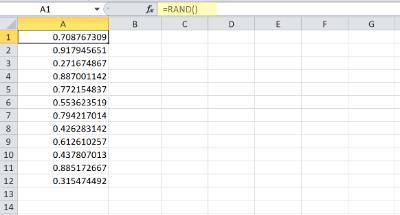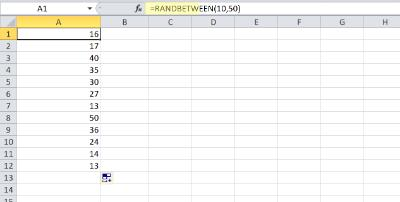# How to generate random number in Excel

15.06.2022

In order to generate a random number in excel, you can use the RAND() function.

Please consider that the RAND() Excel function returns a value between 0 and 1.

You can multiply this value with your required range if you need bigger numbers, or integers. Also, please consider that any recalculation made on your Excel sheet, will refresh the generated random numbers## Random numbers in excel between two limits (min/max)

If you need random numbers between two limits, you can use function RANDBETWEEN(min, max) function in ExcelMicrosoft Documentation about RAND and RANDBETWEEN excel functions

You can read more about RAND and RANDBETWEEN excel functions on Microsoft Documentation here: Microsoft docs